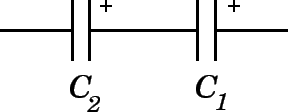# A 3.91 μF capacitor and a 7.41 μF capacitor are connected in series across a 12.0 V battery. What voltage would be required to charge a parallel combination of the same two capacitors to the same total energy?

Aug 2, 2015

$5.7 \text{V}$

#### Explanation:The capacitance $C$ of 2 capacitors in series like this is given by:

$\frac{1}{C} = \frac{1}{C} _ \left(1\right) + \frac{1}{C} _ 2$

This can be rearranged to:

$C = \frac{{C}_{1} {C}_{2}}{{C}_{1} + {C}_{2}}$

$C = \frac{3.91 \times {10}^{- 6} \times 7.41 \times {10}^{- 6}}{\left(3.91 + 7.41\right) \times {10}^{- 6}}$

$C = 2.56 \times {10}^{- 6} \text{F}$

$= 2.56 \mu \text{F}$

The energy of a capacitor is given by:

$E = \frac{1}{2} C {V}^{2}$

$E = \frac{1}{2} \times 2.56 \times {10}^{- 6} \times {12}^{2}$

$E = 1.84 \times {10}^{- 4} \text{J}$

For capacitors in parallel:$C = {C}_{1} + {C}_{2}$

$C = 3.91 + 7.41 = 11.32 \mu \text{F}$

$E = \frac{1}{2} C {V}^{2}$

${V}^{2} = \frac{2 E}{C}$

$= \frac{2 \times 1.84 \times {10}^{- 4}}{11.32 \times {10}^{- 6}}$

${V}^{2} = 32.5$

$V = 5.7 \text{V}$